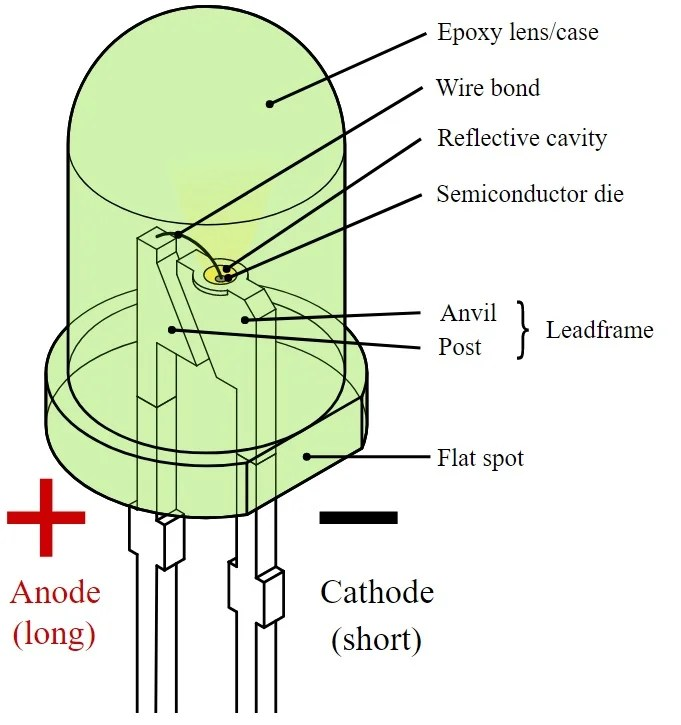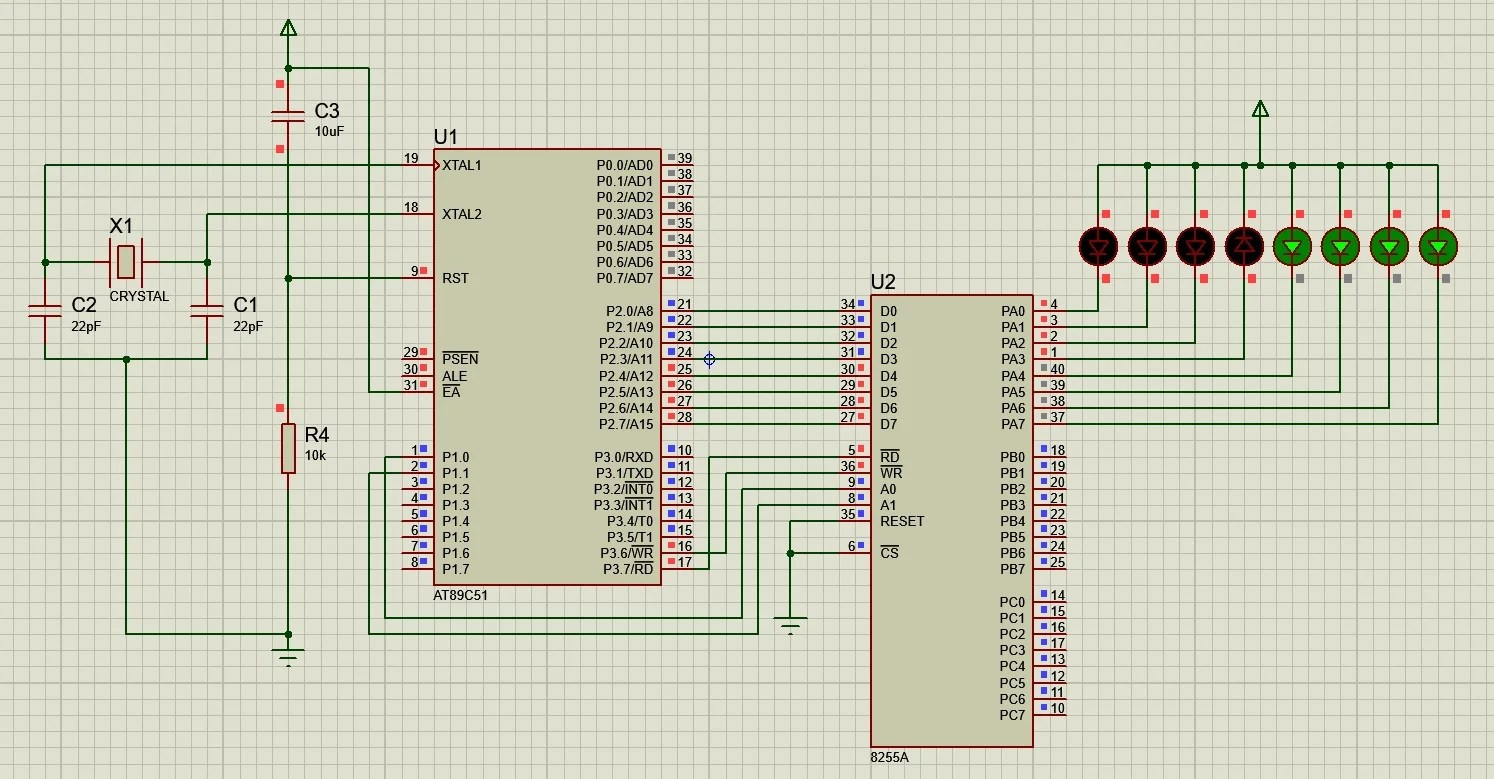View Course Path

# LED interfacing with 8051 – Direct and with 8255

Here’s what we are going to cover in this tutorial:

• Connect an LED to 8051 and make it blink with a preset time interval between LED ON and LED OFF.
• Connect more than one LED to 8051 and program it to work according to our needs and application.
• Use the 8255 PPI to interface 8 LEDs with 8051 (indirect connection) and write a program for it.

Feel free to brush up your concepts of 8255 PPI interfacing with 8051 here. In case you need to understand the working of the 8255 PPI, check out the guide here.

Contents

## Components required for blinking LEDs

1. 8051 microcontroller – AT89C51/AT89S51/52 or similar variants.
2. 8255 PPI
3. LEDs x 8
4. Oscillator crystal – 12Mhz
5. Capacitors – 22pF x 2, 10µF x 1
6. Resistors – 10kΩ x 1, 0.5kΩ x 2

## How do LEDs work?

Light Emitting Diodes or LEDs are the most commonly used components in many applications. They are made of semiconducting material. The principle of working of an LED is similar to that of a diode, where the LED turns ON in forward-bias condition and turns OFF in reverse-bias condition. The anode is the positive terminal, and the cathode is the negative terminal of an LED. When a higher potential is connected to the anode and lower potential is connected to the cathode, the current flows from anode to cathode of LED (higher to lower potential), the LED turns ON and is said to be in forward-bias.## Direct LED interfacing with 8051

To use an LED as the output device, the LED should be connected to an 8051 microcontroller’s port and must be programmed to blink.

### Blinking one LED with the 8051

There are two ways in which we can interface an LED to an 8051. But the connections and programming techniques will be different.• In method 1, the LED will glow only if the PIN value of the 8051 microcontroller is LOW as current flows towards PIN from the +5V supply due to its lower potential.
• In method 2, the LED will glow only if the PIN value of the 8051 microcontroller is HIGH as current flows towards the ground.

The LED is connected to Port 2 (or any other port) of 8051 through a current limiting resistor.

The 8051 microcontroller has four general purposes I/O ports, which can be configured as input or output. Configuring the port pins as output, the state of the port pins can be controlled and set to either high or low. When the port is configured as input, reading the pins will read the voltage state of the pins. We need to configure the port pins as output for the LED blinking process.

### Circuit diagram to directly interface one LED with 8051### Code to directly interface 1 LED with 8051

We generate a nominal delay of 50ms by using a nested for loop. You can check out the tutorial on timers and counters of 8051 to generate a delay of the required time interval using the built-in timers.

Here, we’ve used reg51.h library as we’re using AT89C51/S51 microcontroller. If you’re using AT89C52/S52 microcontroller, just replace `#include<reg.51>` with `#include<reg.52>` and you’re good to go!

```#include<reg51.h>
sbit LED_pin = P2^0; // LED_pin is connected to P2.0

void ms_delay(unsigned int ms) // to provide a delay in ms
{
unsigned int i;
for(i=0;i<=ms;i++);
}

void main()
{
P2 = 0x00; // to make P2 as output port
while(1) // Repeat this loop until stopped
{
LED_pin = 1; // Turn ON the LED
ms_delay(50); // Delay of 50ms
LED_pin = 0; // Turn OFF the LED
ms_delay(50); // Delay of 50ms
}
}```

### Blinking two LEDs with the 8051

Here, to connect more than one LED to 8051, we connect another LED to pin P2.5. In fact, we can connect eight LEDs at once to a single port of 8051. The command `sbit LED_pin` is used to interface the port 2 pins with the LEDs. To blink the LEDs one after the other, we make LED_Pin_1 HIGH for 50ms (by using the `ms_delay()` function), then make it LOW for 50ms. Next, LED_Pin_2 is set to HIGH for 50ms, then turn it OFF for 50ms before turning ON the first LED again.

### Circuit diagram to directly interface two LEDs with 8051### Code to directly interface two LEDs with 8051

• Port 2 pin 0 is declared as LED_pin_1, which means we’re using this bit (microcontroller pin) for the first LED (Green). Hence, the declaring statement will be `sbit LED_pin_1 = P2^0;`
• Port 2 pin 1 is declared as LED_pin_2, which means we’re using this bit (microcontroller pin) for the second LED (Blue). Hence, the declaring statement will be `sbit LED_pin_2 = P2^5;`
```#include<reg51.h>
sbit LED_pin_1 = P2^0;
sbit LED_pin_2 = P2^5;

void ms_delay(unsigned int ms) // to provide a delay in ms
{
unsigned int i;
for(i=0;i<=ms;i++);
}

void main()
{
P2 = 0x00; // to make P2 as output port
while(1) // Repeat this loop until stopped
{
LED_pin_1 = 1; // Turn ON the LED 1
ms_delay(50); // Delay of 50ms
LED_pin_1 = 0; // Turn OFF the LED 1
ms_delay(50); // Delay of 50ms

LED_pin_2 = 1; // Turn ON the LED 2
ms_delay(50); // Delay of 50ms
LED_pin_2 = 0; // Turn OFF the LED 2
ms_delay(50); // Delay of 50ms
}
}```

## Indirect LED interfacing with 8051 using 8255 Programmable Peripheral Interface

Before we use the 8255 Programmable Peripheral Interface, I would recommend you check out it’s working, pin diagram, modes, how to select the ports (A, B, C, and the control register) and how to construct the control word for the control register: 8255 Programmable Peripheral Interface – PPI guide.

Here, we’ll use the Port A of 8255 in mode 0 by programming it using pin P1.0 and P1.1 of 8051.

### Circuit Diagram

• Step 1: If you’re using Proteus or and other simulation software or even hardware, select the AT89C51 or AT89S51 microcontroller or any other compatible variant. (The AT89C51 is an 8-bit microcontroller from the Atmel family which works with the 8051 architecture.)
• Step 2: Connect a 12 MHz oscillator between pin 18 and 19.
• Step 3: Connect two capacitors of 22pF, with one terminal on either side of the oscillator and the other terminal to ground, as shown below.
• Step 4: Set Pin 31, i.e., EA pin to HIGH by connecting it to the +5V DC source.
• Step 5: Now, to make the RESET circuit, connect Pin 9 (RST) to +5V through a capacitor of 10µF and connect the same pin to +0V (GND) through a 10kΩ resistor or a potentiometer.
• Step 6: Connect WR and RD of 8255 PPI to P3.6 and P3.7, respectively, of 8051.
• Step 7: Connect Port 2 (P2.0 – P2.7) to data pin (D0 – D7), respectively.
• Step 8: Connect CS, RESET, GND to ground, and VCC to +5V supply.
• Step 9: Connect A0 and A1 of 8255 PPI to P1.0 and P1.1, respectively, of 8051.
• Step 10: The final step is to connect PA0 – PA7 to cathode LEDs and anode to +5V VCC.### C code to interface LEDs using 8255 PPI with 8051

We start off by making Port 1, 2, and 3 as output ports by sending 00H to it. Then we disable RD and enable WR by sending 1 and 0 respectively as they are active low pins (Turns ON when a low pulse is provided). The next step is to select mode 0 of 8255 by sending 0 to A1 and A0 pins.

Before we send the pulses to make the LEDs glow, we must make the Ports of 8255 as output ports by sending 1000000 through port 2 to 8255. Next up is the logic of the pattern in which we make the LEDs blink. First, we’ll blink the LEDs connected to the lower nibble then the LEDs connected to the higher nibble with a delay in between to make sure the “blinking” is visible.

```#include<reg52.h>
sbit A0 = P1^0;
sbit A1 = P1^1;
sbit wr = P3^6;
sbit rd = P3^7;

void delay(unsigned int ms) // to provide a delay in ms
{
unsigned int i;
for(i=0;i<=ms;i++);
}

void main()
{
P1 = 0x00; // Port-1 as Output
P2 = 0x00; // Port-2 as Output
P3 = 0x00; // Port-3 as Output
delay (10000); // Delay before starting to write

rd = 1; // Disabling read from
wr = 0; // Enabling write to
P2 = 0x80; // Making all ports output
A0 = 1; // Selecting control reg
A1 = 1; // Selecting control reg

while(1)
{
wr = 1;
A0 = 0; // Select Port A as output
A1 = 0;
delay (10000); // Sufficient delay is to be provided to see the blinking
P2 = 0x0F; // To make LEDs connected to higher nibble glow as we’re using method 1
wr = 0;
// You can also write P2 = ~0x0F to make LEDs connected to lower nibbles light up.

wr = 1;
A0 = 0; // Select Port A as output
A1 = 0;
delay (10000); // Sufficient delay is to be provided to see the blinking
P2 = 0xF0; // To make LEDs lower nibble light up as we’re using method 1
wr = 0;
}
}```

## Application of LEDs

• They are used in indicator circuits, like a water level sensor, to indicate that the water may overflow or temperature sensors circuits.
• Used in chargers, mobile phones, every other device to indicate whether the power supply to the particular device is present or not.
• They are the building blocks of seven-segment displays and LCD modules.

This site uses Akismet to reduce spam. Learn how your comment data is processed.